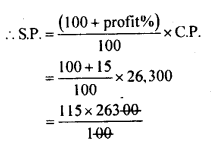# KSEEB Solutions for Class 8 Maths Chapter 9 Commercial Arithmetic Ex 9.6

Students can Download Maths Chapter 9 Commercial Arithmetic Ex 9.6 Questions and Answers, Notes Pdf, KSEEB Solutions for Class 8 Maths helps you to revise the complete Karnataka State Board Syllabus and score more marks in your examinations.

## Karnataka Board Class 8 Maths Chapter 9 Commercial Arithmetic Ex 9.6

Question 1.
A person purchases the following items from a mall for which the sales tax is mentioned against.
(a) Stationary materials for Rs. 250 and sales tax of 4% there on.
(b) Electronic goods worth Rs. 2580 and sales tax of 10% there on.
(c) Groceries worth Rs. 1200 on which sales tax of 3% is levied.
(d) Medicines worth Rs. 200 with sales tax of 6%. Find the bill amount for each item.

(a) Marked price Rs. 250, and sales tax 4%
Amount to be paid = marked price + tax
TaxBill amount = 250+10 = Rs. 260.

(b) Marked price is Rs. 2580 and sale tax 10%
Tax =Bill amount = 2580 + 258 = Rs. 2838.

(c) Marked price Rs. 1200 and tax 3%
Tax =Bill amount = 1200 + 36 = Rs. 1236.

(d) Marked price Rs. 200 and sales tax 6%
Tax =Bill amount = 200 + 12 = Rs. 212.

Question 2.
A person buys electronic goods worth Rs. 10,000 for which the sales tax is 4% and other material worth Rs. 15,000 for which the sales tax is 6%. He manufactures a gadget using all these and sells it at 15% profit, what is his selling price?
Price of the electronic goods is Rs. 10,000 and tax is 4%
∴ Tax =$$\frac { 4 }{ 100 }$$ × 10,000 = Rs.400
Total cost = 10,000 + 400 = Rs. 10,400
Price of the other material is 15,000 and tax is 6%.
∴ Tax = $$\frac { 6 }{ 100 }$$ × 15,000 = Rs.900
Total cost = 15,000 + 900 = Rs. 15,900
Total cost price of the electronic gadget prepared by him is (10400 + 15900) = 26300
Profit percent = 15%selling price = Rs. 30,245

Question 3.
A trader purchases 70 kg of tea at the rate of Rs. 200 kg and another 30kg at the rate of Rs. 250/kg. He pays a sales tax of 4% on the transaction. He mixes both of them and sells the product at the rate of Rs. 240/kg. What is the percentage gain or loss (find approximate value)?
The cost of 70 kg of tea at the rate Rs. 200/ kg is 200 x 70 = Rs. 14000
The cost of 30kg of tea at the rate Rs 250/ kg is 250 x 30 = Rs 75000.
Total cost of 100kg = 14000+7500 = Rs. 21,500
Rate of tax 4%
∴ Tax = $$\frac { 4 }{ 100 }$$ × 21,5000 = Rs.860
Total cost price = 21,500 + 860 = Rs. 22,360
S.P of 100kg tea at Rs. 240/Kg
= 100 x 240 = Rs. 24,000
S.P > C.P
Profit = S.P – C.P
= 24,000 – 22360 = Rs. 1640Profit percent = 7.33%

error: Content is protected !!